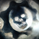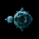4095 views
4095
Squeeze Momentum Indicator - Optimized

All Updates from Version 3 + Squeeze Optimized further.
// Heikin Ashi Optimization Applied

study(shorttitle = "UCS_SQZ_Opt", title="UCS_Squeeze Momentum - Optimized", overlay=false)

length = input(20, title="Squeeze Length")
multBB = input(2,title="BB MultFactor")
multKC = input(1.5, title="KC MultFactor")
smooth = input(20, title = "Momentum Smoothing")

usebbr = input(true, title = "Use Bollinger Band Ratio Instead of Momentum", type = bool)
useHAC = input(true, title = "Heikin Ashi Optimization", type=bool)

// Heikin Ashi ATR Calculations
haclose = ohlc4
haopen = na(haopen) ? (open + close)/2 : (haopen + haclose) / 2
hahigh = max (high, max(haopen,haclose))
halow = min (low, min(haopen,haclose))
haatra = abs(hahigh - haclose)
haatrb = abs(haclose - halow)
haatrc = abs(hahigh - halow)
haatr = max(haatra, max(haatrb,haatrc))

source = useHAC ? haclose : close

// Calculate BB
basis = sma(source, length)
dev = multBB * stdev(source, length)
upperBB = basis + dev
lowerBB = basis - dev

// Calculate KC
ma = sma(source, length)
range = useHAC ? haatr : tr
rangema = sma(range, length)
upperKC = ma + rangema * multKC
lowerKC = ma - rangema * multKC

sqzOn  = (lowerBB > lowerKC) and (upperBB < upperKC)
sqzOff = (lowerBB < lowerKC) and (upperBB > upperKC)
noSqz  = (sqzOn == false) and (sqzOff == false)

// Momentum ======> %B Indicator OR Rate of Change (ROC)
momentum = usebbr ? (((source - lowerBB)/(upperBB - lowerBB))-0.5) : (((close - close)/close)*100)
val = sma(momentum,smooth)

// Plot Statements
bcolor = iff( val > 0,
iff( val > nz(val), green, blue),
iff( val < nz(val), red, orange))
scolor = noSqz ? blue : sqzOn ? red : green
plot(val, color=bcolor, style=histogram, linewidth=3)
plot(0, color=scolor, style=circles, linewidth=3)Hello, I just joined so not sure how active this thread is. I'm very interested in using the 'Squeeze event' for trading...I have access to TTM_Squeeze on ThinkorSwim. But I'll be interested if I can try your versions using this sites charts. Is there by chance a way to use your Squeeze indicator on the stock screener to find stocks that fit a breakout scenario. Actually I'm interested in a different use. I would like to be able to scan for stocks that on the Weekly chart data signal a first red dot(begin of squeeze) with a few other conditions; above 52sma, price > \$40, and large trade volume.
Is there any way I could use your indicator to scan for such stocks?
Is there an easy way for your code to be translated into Thinkscript for TOS?
Thanks
ReplyNeoFitz
There is no way to scan for stocks in Tradingview, Yet. TOS have scanners. The codes from TV can be translated. Provided you can convert them, Its simple.
ReplyThere are sooo many ways this tool can be used! Here I simply overlapped a longer time frame (weekly) momentum over the one day momentum. This tool makes spotting divergence extremely easy.

ReplyAlphaoptions
thanks ! just like to help with what I see, what I know, UCS GEARS puts up great tools. I can go on and on and on, but I wouldnt want to hi-jack the thread.
Replyucsgears
You are very welcome, and thank you for your generosity !
Reply